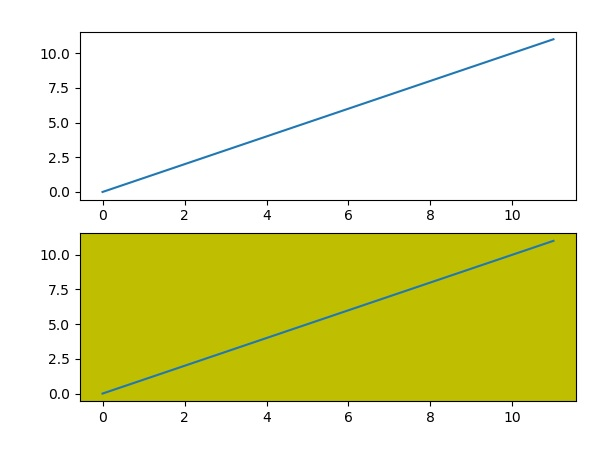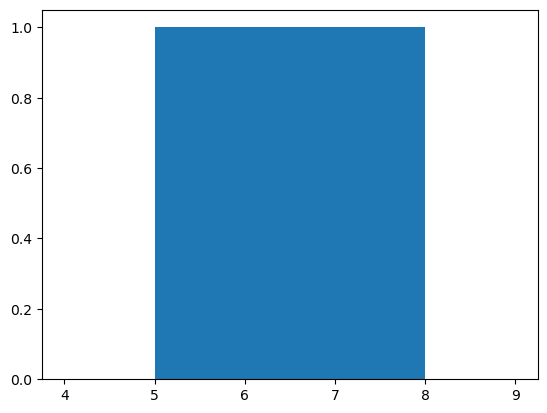python list append multiple times

# Matplotlib for Data Visualization python

Introduction

Want to learn Data Visualization using Matplotlib?
Hello friends, in the previous blog post we have to see basic pandas library Numpy and pandas so it’s time to see Data viasualization in python using Matplotlib. so first upon what is matplotlib ? let’s start. Matplotlib is the grandfathers library of data visualizations with Python. It was created by the John Hunter. He created it to try to replicate MatLab(another programming language) plotting capabilities in Pythons. So if you happen to be familiar with matlab, matplotlibs will feel natural to you.

Also read : scope in python
It is an excellent 2D and 3D graphic library for generating scientific figures.data visualization with matplotlib using python from zero to hero fig 01)Matplotlib for Data Visualization python

Some of the major Pros of Matplotlibs are:

1. Generally easy to started for simple plot
2. Support for custom labels and text
3. Great control of every element in a figures
4. High-quality output in many formats
5. Very customizable in general
Matplotlib allows you to create reproducible figures programmatically. Let’s learn how to use it! Before continuing this lecture, I encourage you just to explore the official Matplotlib web page: http://matplotlib.org/

## Installation

You’ll need to install matplotlib first with either:
``conda install matplotlib``
or pip install matplotlib

5.1 example:

Creating a subplot will delete any pre-existing subplots that overlaps with it beyond sharing a boundary.

import matplotlib.pyplot as plt

import matplotlib.pyplot as plt

# plot a lines, implicitly creating a subplot(111)

plt.plot([1,2,3])

# now create a subplots which represents the top plot of a grid with 2 rows and 1 column.

#Since this subplots will overlap the first, the plot (and its axes) previously

created, will be removed

plt.subplot(211)

plt.plot(range(12))

plt.subplot(212, facecolor=’y’) # create 2nd subplots with yellow background

plt.plot(range(12))

The above line produce the following outputs −data visualization with matplotlib using python from zero to hero

5.2 Example:

Following code plots a histogram of marks obtained by students in class. Four bins, 0-25, 26-50, 51-74 are defined.

from matplotlib import pyplot as plt

import numpy as np

fig,ax = plt.subplots(1,1)

a = np.array([22,87,5,43,56,73,55,54,11,20,51,5,79,31,27])

ax.hist(a, bins = [0,25,50,75,100])

ax.set_title(“histogram of result”)

ax.set_xticks([0,25,50,75,100])

ax.set_xlabel(‘marks’)

ax.set_ylabel(‘no. of students’)

plt.show()

The plot appears as shown below −data visualization with matplotlib using python from zero to hero

Summary :

In this article we saw data visualization with matplotlib using python from zero to hero so about this section you have any problem then free to ask me

BEST OF LUCK !!!

### sachin Pagar

I am Mr. Sachin pagar Embedded software engineer, the founder of Sach Educational Support(Pythonslearning), a Passionate Educational Blogger and Author, who love to share the informative content on educational resources.

### This Post Has 4 Comments

1.Anonymous

Nice